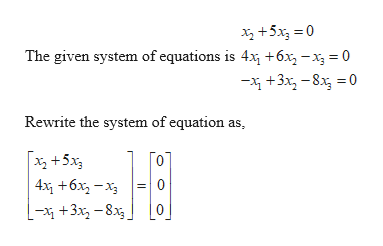# write a vector equation that is equivalent to the given system of equations. x2 + 5x3= 0 4x1 + 6x2– x3 = 0–x1+ 3x2– 8x3= 0

Question
23 views

write a vector equation that is equivalent to the given system of equations. x2 + 5x3= 0

4x1 + 6x2– x3 = 0

–x1+ 3x2– 8x3= 0

check_circle

Step 1help_outlineImage TranscriptioncloseXz +5x, = 0 The given system of equations is 4x, +6x, -x = 0 -x +3x, -8x, = 0 Rewrite the system of equation as, x +5x 4x, +6x, - x3 [-x +3x -8x, fullscreen

### Want to see the full answer?

See Solution

#### Want to see this answer and more?

Solutions are written by subject experts who are available 24/7. Questions are typically answered within 1 hour.*

See Solution
*Response times may vary by subject and question.
Tagged in

### Other